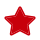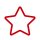# SQL优化

#### 样例

```select
sum(l_extendedprice* (1 - l_discount)) as revenue
from
lineitem,
part
where
(
p_partkey = l_partkey
and p_brand = 'Brand#32'
and p_container in ('SM CASE', 'SM BOX', 'SM PACK', 'SM PKG')
and l_quantity >= 7 and l_quantity <= 7 + 10
and p_size between 1 and 5
and l_shipmode in ('AIR', 'AIR REG')
and l_shipinstruct = 'DELIVER IN PERSON'
)
or
(       p_partkey = l_partkey
and p_brand = 'Brand#35'
and p_container in ('MED BAG', 'MED BOX', 'MED PKG', 'MED PACK')
and l_quantity >= 15 and l_quantity <= 15 + 10
and p_size between 1 and 10
and l_shipmode in ('AIR', 'AIR REG')
and l_shipinstruct = 'DELIVER IN PERSON'
)
or
(       p_partkey = l_partkey
and p_brand = 'Brand#24'
and p_container in ('LG CASE', 'LG BOX', 'LG PACK', 'LG PKG')
and l_quantity >= 26 and l_quantity <= 26 + 10
and p_size between 1 and 15
and l_shipmode in ('AIR', 'AIR REG')
and l_shipinstruct = 'DELIVER IN PERSON'
)```

```select
sum(l_extendedprice* (1 - l_discount)) as revenue
from
lineitem,
part
where   p_partkey = l_partkey and
((
p_brand = 'Brand#32'
and p_container in ('SM CASE', 'SM BOX', 'SM PACK', 'SM PKG')
and l_quantity >= 7 and l_quantity <= 7 + 10
and p_size between 1 and 5
and l_shipmode in ('AIR', 'AIR REG')
and l_shipinstruct = 'DELIVER IN PERSON'
)
or
(
p_brand = 'Brand#35'
and p_container in ('MED BAG', 'MED BOX', 'MED PKG', 'MED PACK')
and l_quantity >= 15 and l_quantity <= 15 + 10
and p_size between 1 and 10
and l_shipmode in ('AIR', 'AIR REG')
and l_shipinstruct = 'DELIVER IN PERSON'
)
or
(
p_brand = 'Brand#24'
and p_container in ('LG CASE', 'LG BOX', 'LG PACK', 'LG PKG')
and l_quantity >= 26 and l_quantity <= 26 + 10
and p_size between 1 and 15
and l_shipmode in ('AIR', 'AIR REG')
and l_shipinstruct = 'DELIVER IN PERSON'
))```

# 相关产品